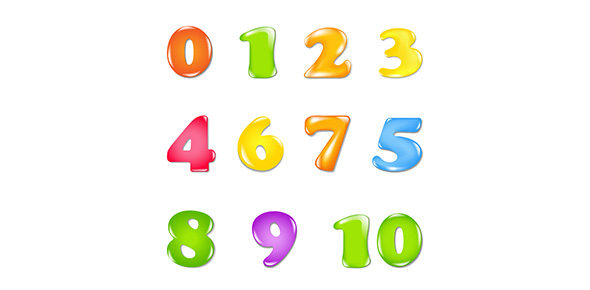# Assigning Oxidation Numbers

10 Questions | Total Attempts: 7624Settings• 1.
What is the oxidation number on N in Li3N?
• 2.
What is the oxidation number on N in N2O5?
• 3.
What is the oxidation number on Hydrogen?
• 4.
What is the oxidation number on N in HNO3?
• 5.
What is the oxidation number for Cu in CuSO4?
• 6.
What is the oxidation number for I2?
• 7.
What is the oxidation number for Mn in MnCl2?
• 8.
What is the oxidation number on Cr4+?
• 9.
What is the oxidation number on F in IF7?
• 10.
The sum of all oxidation numbers must equal?
Related TopicsBack to top